# RS Aggarwal Solutions for Class 10 Maths Chapter 17 Volume and Surface Area of Solids Exercise 17D

R S Aggarwal solutions are given here contain answers to all the questions enlisted in the exercise 17D. Students should go through the R S Aggarwal Solutions for Class 10 Maths exercise 17d to learn how to find volume and surface areas of geometrical figures. These solutions are prepared by subject experts at BYJU’S as per CBSE latest syllabus. Practice R S Aggarwal Maths Chapter 17 for Class 10 effectively to score good marks in the examinations.

## Download PDF of RS Aggarwal Solutions for Class 10 Maths Chapter 17 Volume and Surface Area of Solids Exercise 17D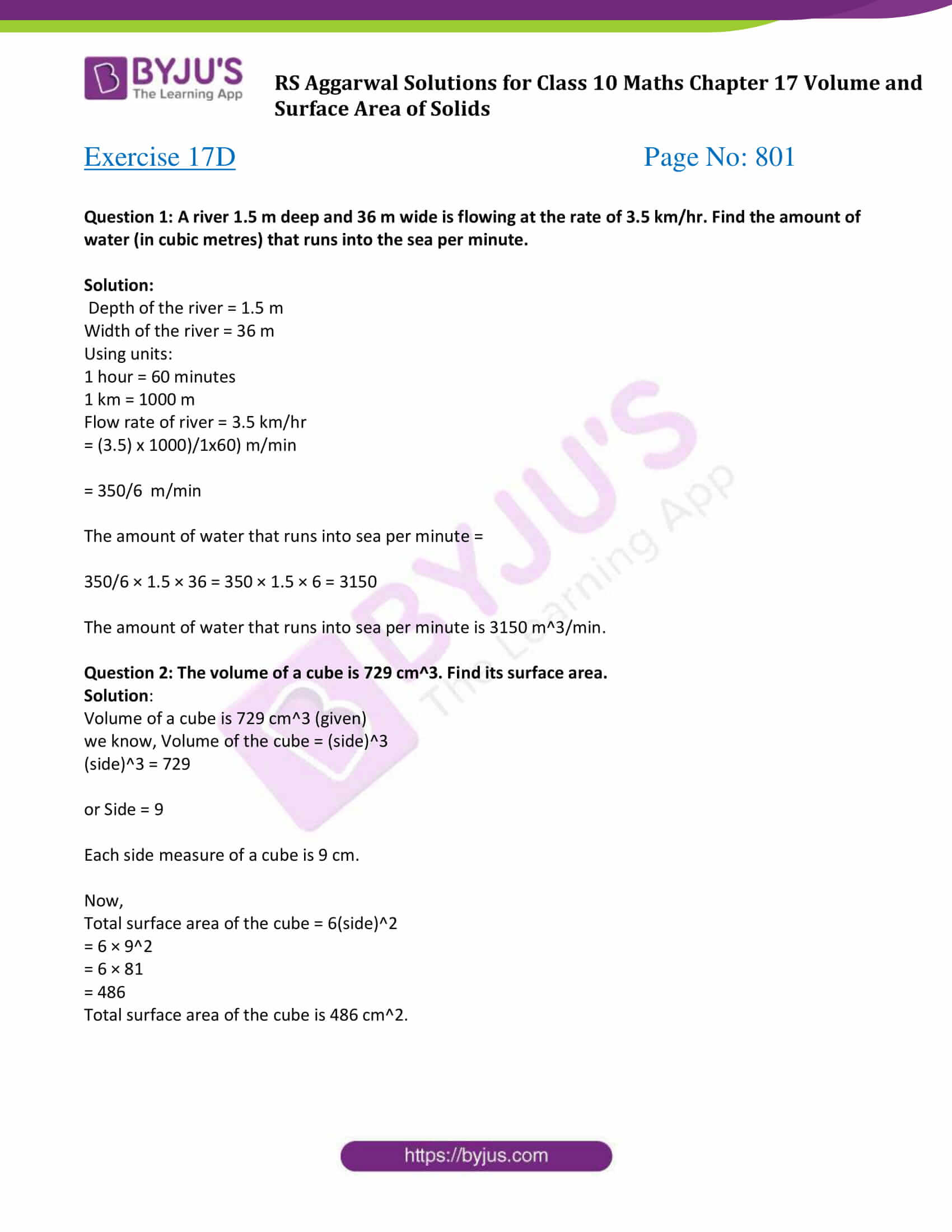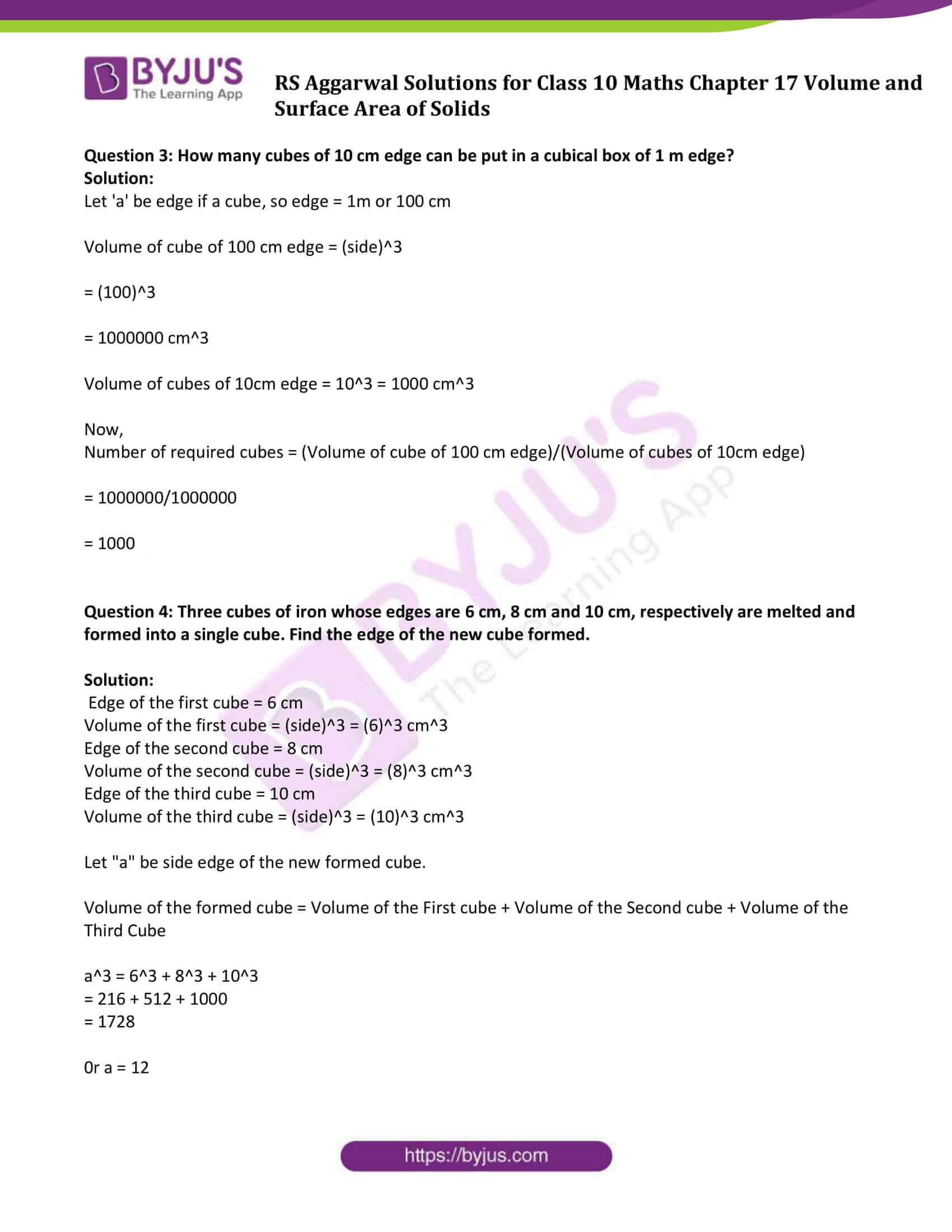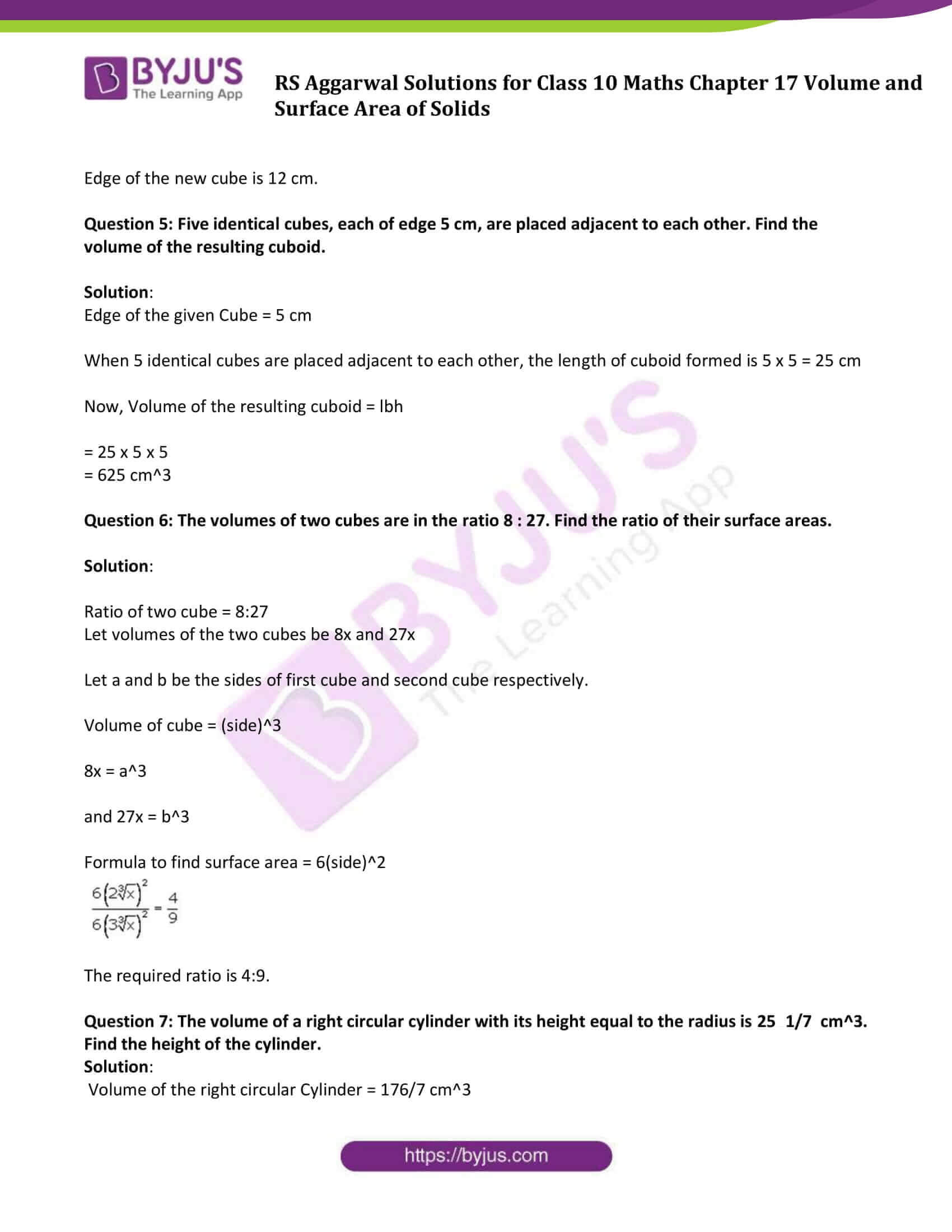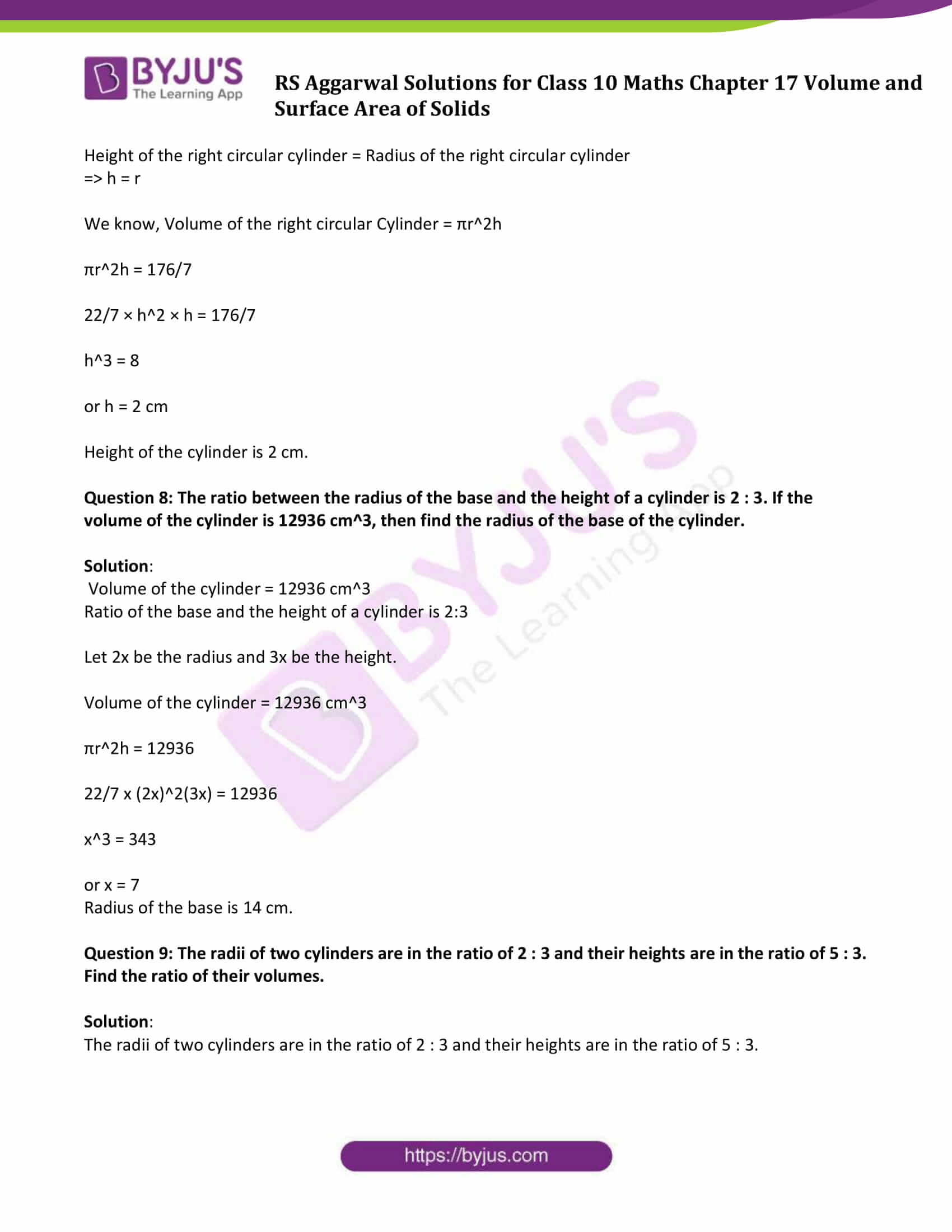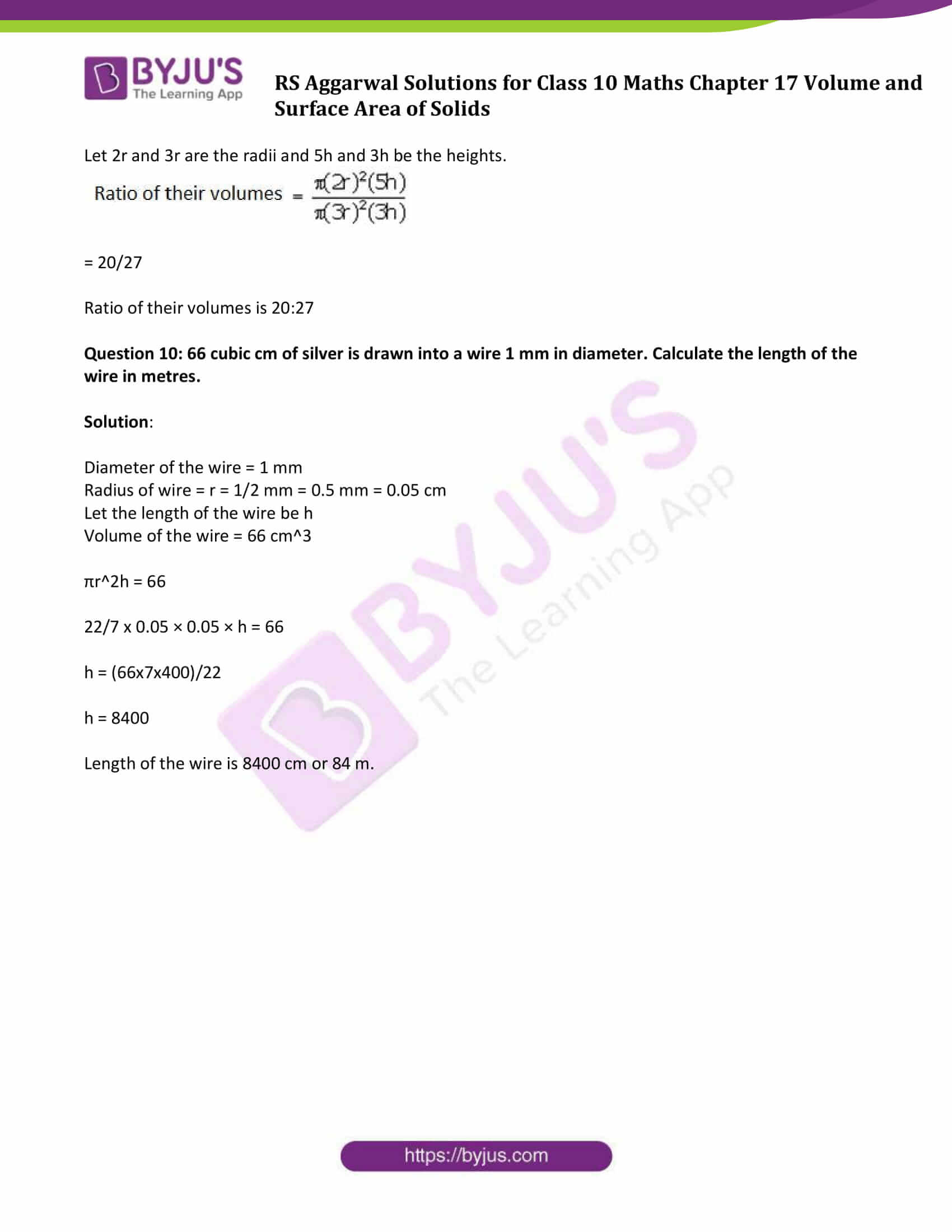### Access other exercise solutions of Class 10 Maths Chapter 17 Volume and Surface Area of Solids

Exercise 17 A Solutions

Exercise 17 B Solutions

Exercise 17 C Solutions

### Access Solutions of Maths RS Aggarwal Class 10 Chapter 17 – Volume and Surface Area of Solids Exercise 17D

Question 1: A river 1.5 m deep and 36 m wide is flowing at the rate of 3.5 km/hr. Find the amount of water (in cubic metres) that runs into the sea per minute.

Solution:

Depth of the river = 1.5 m

Width of the river = 36 m

Using units:

1 hour = 60 minutes

1 km = 1000 m

Flow rate of river = 3.5 km/hr

= (3.5) x 1000)/1×60) m/min

= 350/6 m/min

The amount of water that runs into sea per minute =

350/6 × 1.5 × 36 = 350 × 1.5 × 6 = 3150

The amount of water that runs into sea per minute is 3150 m3/min.

Question 2: The volume of a cube is 729 cm3. Find its surface area.

Solution:

Volume of a cube is 729 cm3 (given)

we know, Volume of the cube = (side)3

(side)3 = 729

or Side = 9

Each side measure of a cube is 9 cm.

Now,

Total surface area of the cube = 6(side)2

= 6 × 92

= 6 × 81

= 486

Total surface area of the cube is 486 cm2.

Question 3: How many cubes of 10 cm edge can be put in a cubical box of 1 m edge?

Solution:

Let ‘a’ be edge if a cube, so edge = 1m or 100 cm

Volume of cube of 100 cm edge = (side)3

= (100)3

= 1000000 cm3

Volume of cubes of 10cm edge = 103 = 1000 cm3

Now,

Number of required cubes = (Volume of cube of 100 cm edge)/(Volume of cubes of 10cm edge)

= 1000000/1000000

= 1000

Question 4: Three cubes of iron whose edges are 6 cm, 8 cm and 10 cm, respectively are melted and formed into a single cube. Find the edge of the new cube formed.

Solution:

Edge of the first cube = 6 cm

Volume of the first cube = (side)3 = (6)3 cm3

Edge of the second cube = 8 cm

Volume of the second cube = (side)3 = (8)3 cm3

Edge of the third cube = 10 cm

Volume of the third cube = (side)3 = (10)3 cm3

Let “a” be side edge of the new formed cube.

Volume of the formed cube = Volume of the First cube + Volume of the Second cube + Volume of the Third Cube

a3 = 63 + 83 + 103

= 216 + 512 + 1000

= 1728

0r a = 12

Edge of the new cube is 12 cm.

Question 5: Five identical cubes, each of edge 5 cm, are placed adjacent to each other. Find the volume of the resulting cuboid.

Solution:

Edge of the given Cube = 5 cm

When 5 identical cubes are placed adjacent to each other, the length of cuboid formed is 5 x 5 = 25 cm

Now, Volume of the resulting cuboid = lbh

= 25 x 5 x 5

= 625 cm3

Question 6: The volumes of two cubes are in the ratio 8 : 27. Find the ratio of their surface areas.

Solution:

Ratio of two cube = 8:27

Let volumes of the two cubes be 8x and 27x

Let a and b be the sides of first cube and second cube respectively.

Volume of cube = (side)3

8x = a3

and 27x = b3

Formula to find surface area = 6(side)2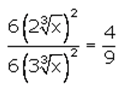The required ratio is 4:9.

Question 7: The volume of a right circular cylinder with its height equal to the radius is 25 1/7 cm3. Find the height of the cylinder.

Solution:

Volume of the right circular Cylinder = 176/7 cm3

Height of the right circular cylinder = Radius of the right circular cylinder

⇨ h = r

We know, Volume of the right circular Cylinder = πr2h

πr2h = 176/7

22/7 × h2 × h = 176/7

h3 = 8

or h = 2 cm

Height of the cylinder is 2 cm.

Question 8: The ratio between the radius of the base and the height of a cylinder is 2 : 3. If the volume of the cylinder is 12936 cm3, then find the radius of the base of the cylinder.

Solution:

Volume of the cylinder = 12936 cm3

Ratio of the base and the height of a cylinder is 2:3

Let 2x be the radius and 3x be the height.

Volume of the cylinder = 12936 cm3

πr2h = 12936

22/7 x (2x)2(3x) = 12936

x3 = 343

or x = 7

Radius of the base is 14 cm.

Question 9: The radii of two cylinders are in the ratio of 2 : 3 and their heights are in the ratio of 5 : 3. Find the ratio of their volumes.

Solution:

The radii of two cylinders are in the ratio of 2 : 3 and their heights are in the ratio of 5 : 3.

Let 2r and 3r are the radii and 5h and 3h be the heights.

= 20/27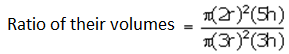Ratio of their volumes is 20:27

Question 10: 66 cubic cm of silver is drawn into a wire 1 mm in diameter. Calculate the length of the wire in metres.

Solution:

Diameter of the wire = 1 mm

Radius of wire = r = 1/2 mm = 0.5 mm = 0.05 cm

Let the length of the wire be h

Volume of the wire = 66 cm3

πr2h = 66

22/7 x 0.05 × 0.05 × h = 66

h = (66x7x400)/22

h = 8400

Length of the wire is 8400 cm or 84 m.

## RS Aggarwal Solutions for Class 10 Maths Chapter 17 Volume and Surface Area of Solids Exercise 17D Topics:

Class 10 Maths Chapter 17 Volume and Surface Area of Solids Exercise 17D is based on the topics:

• Volume and Surface Area of Cuboid
• Volume and Surface Area of Cube
• Volume and Surface Area of cone
• Volume and Surface Area of cylinder and hollow cylinder
• Volume and Surface Area of a frustum of a cone